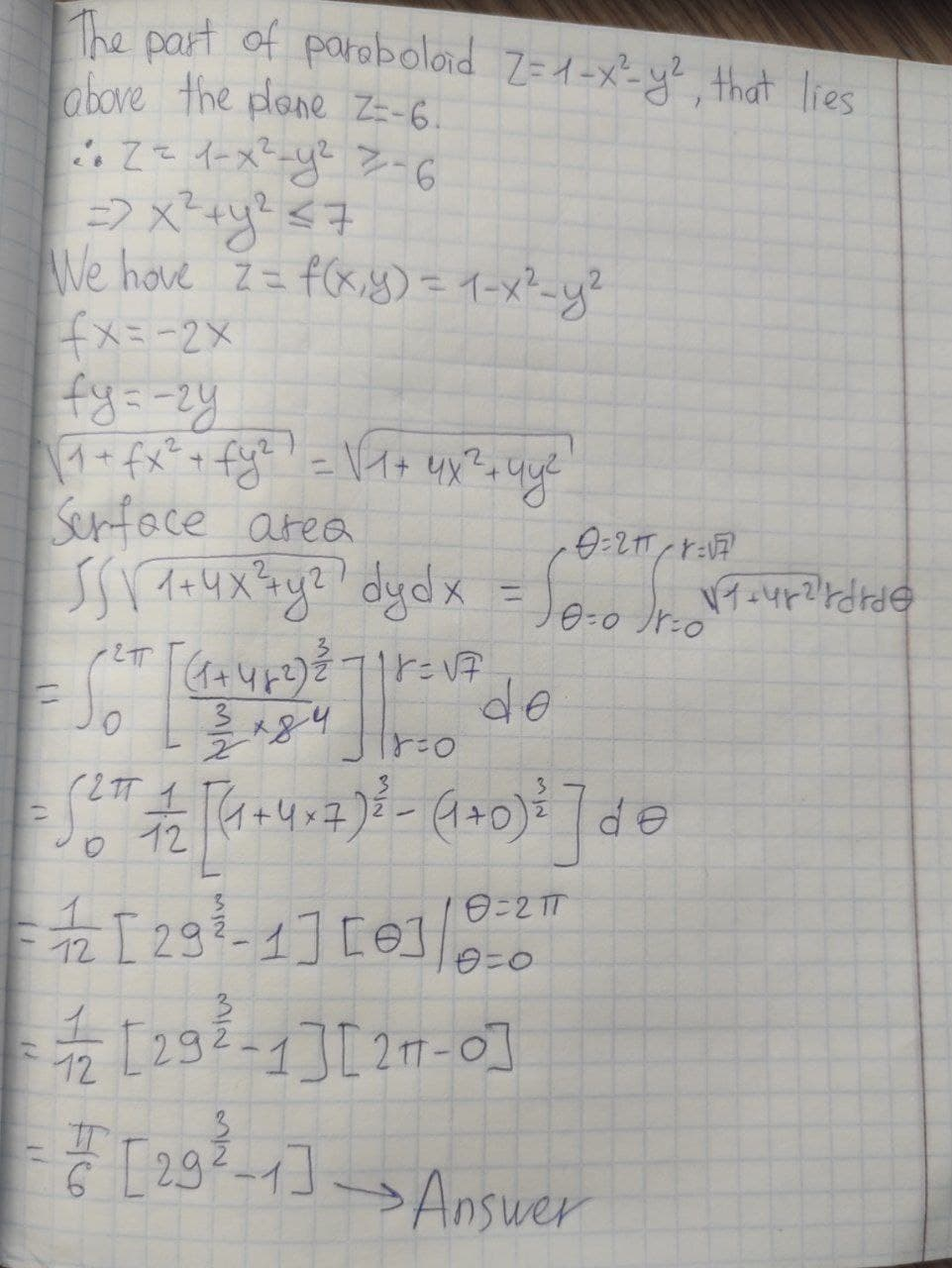# Find the area of the surface.The part of the paraboloid z = 1 − x^2 − y^2 that lies above the plane z = -6generals336 2021-09-07 Answered
Find the area of the surface.
The part of the paraboloid
$z=1-{x}^{2}-{y}^{2}$
that lies above the plane
$z=-6$
You can still ask an expert for help

• Questions are typically answered in as fast as 30 minutes

Solve your problem for the price of one coffee

• Math expert for every subject
• Pay only if we can solve itFaiza Fuller